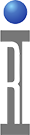The RI7100 can be configured with a two channel digitizer, in the WF6 and WF7 pogo locations.

Basic Specifications:
WF6 : Channel 1
WF7 : Channel 2
Max input : 0.4 V peak
Input impedance : 10K ohm in series with 30pF(testhead cabling); plus an additional 30pF typical for fixture cabling.
Amplitude resolution: >12 bits
Repetitive BW : 100 MHz Max
Digitization Rate : 100 kHz Max

The digitizer works using an under-sampling technique to capture a waveform. Because of this the exact frequency of the waveform must be known to avoid aliasing affects. If the desired measurement is frequency and it is unknown, RF4 is the appropriate tester resource in most cases.

The buttons that control the digitizer are input frequency, time per division and samples. It helps to envision an oscilloscope trace to understand the buttons. The input frequency is the frequency of the waveform being measured. It needs to be exact.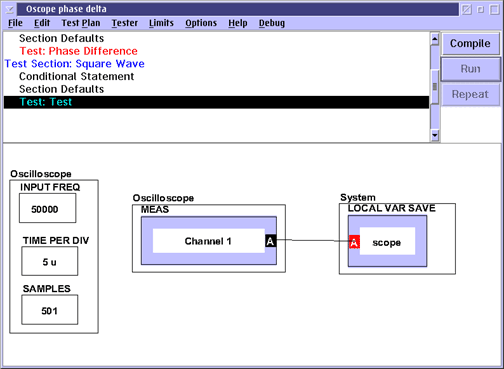Displaying results in Real Time:

The time per division is 1/10th of the "length" of the wave form to be acquired. A good rule of thumb for initial measurement settings is to make the time per division equal to the inverse of frequency. In that case you will capture 10 periods of the waveform (due to 10 divisions on the display).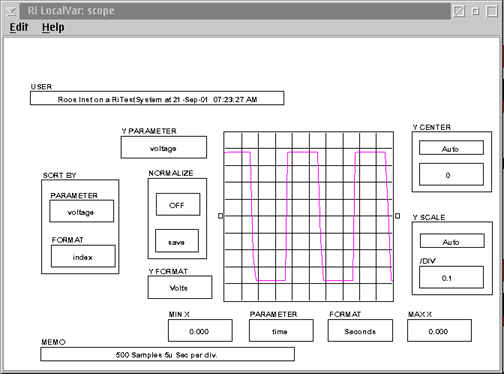The last button is samples. That is the number of points spread across the 10 divisions. 201 samples will generate 20 points per period of the waveform.

The optimum conditions for the time per division and number of samples depends upon the specific measurement. For instance, measurements of rise and fall time will require many samples on few periods of the waveform for efficient measurements.

Interfacing:
The digitizers maximum input signal level is 0.4 V peak. As such, any larger signals need to be attenuated for the 0.4 V maximum rating.

In addition care must be taken to make sure that the digitizer impedance doesn't limit the bandwidth of the required measurement. Let us consider the case of a 3V signal that needs to be measured with the digitizer.

First we need to divide the circuit to get a 0.4V maximum signal. A simple resistive divider will suffice. Putting a 90K Ohm resistor in series with the 20 K ohm impedance of the digitizer reults in a 10:1 divide ratio.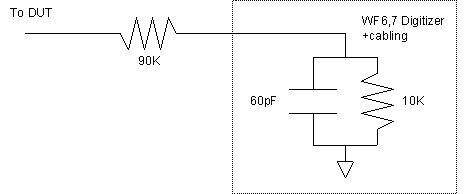But care must be taken to compensate for the timeconstant of the RC network. In this case a compensation capacitance of 6.7 pF will result in an equivalent time constant.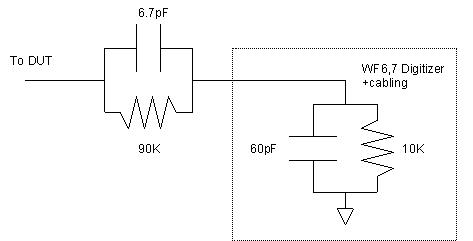https://roos.com/docs/MFEY-52QQYG
ROOS INSTRUMENTS CONFIDENTIAL AND PROPRIETARY